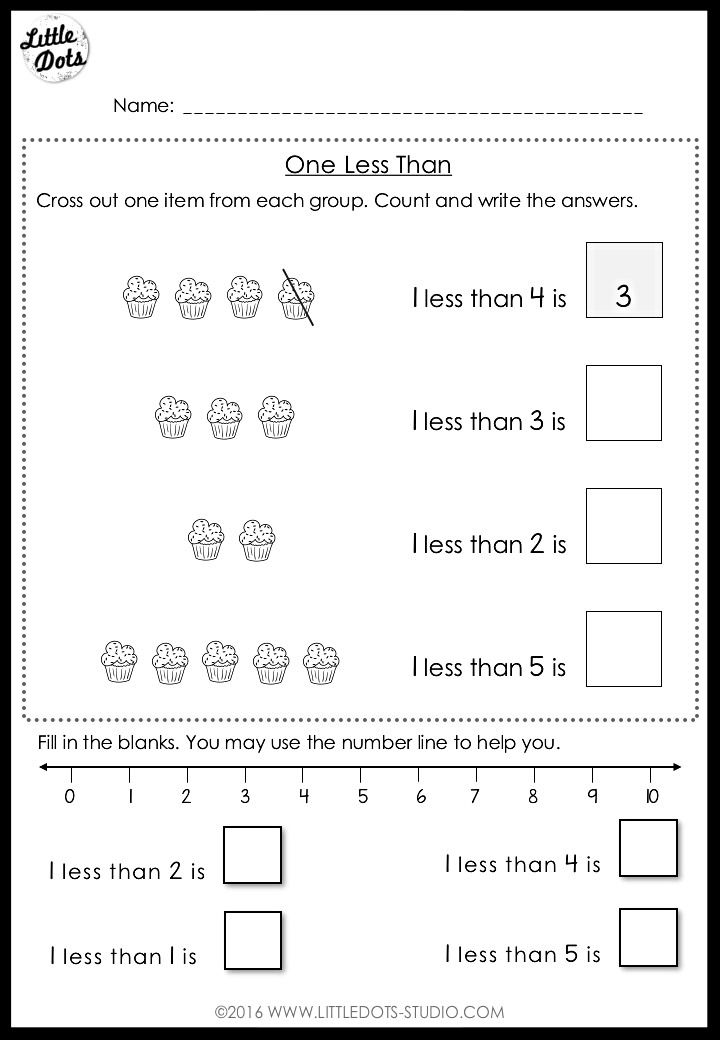top of pageSearch

# Kindergarten Subtraction Worksheets and Activities

Updated: Jan 31, 2022

Today, I have a set of subtraction worksheets and activities for kindergarten level. This set includes 18 subtraction worksheets within 10 to help your children:

• Understand the relationships between 'taking away' and 'less'

• Practice solving subtraction equations within 10 through elimination (crossing out)

• Learn using number line to solve subtraction problems

• Practice to write out subtraction equations from visuals (i.e. objects, fingers, ten frames)

• Create own subtraction equations

• 6 worksheets to help children understand the relationships between 'taking away' and 'less', perfect for introduction to addition. Check out some of the sample worksheets below.• 2 worksheets to practice solving subtraction equations with objects• 4 worksheets to practice writing out subtraction equations• 2 worksheets to create own subtraction equations​• 2 worksheets to practice solving subtraction equations using number lineClick on the link below to get a copy of the subtraction bundle set.